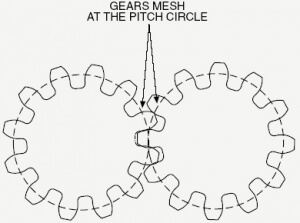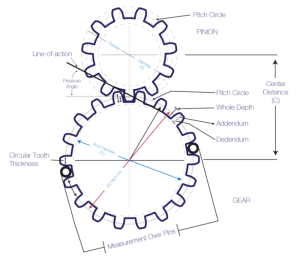# Understanding Gear Profile and Gear Module

The geometry of a simple spur gear tooth is complex. Numerous dimensional parameters define the shape of the tooth profile, determine how the gears fit together, and identify locations where forces act at the gear teeth.  Two of the most important parameters, often confused with each other, are the pitch diameter (or thread pitch diameter), typically denoted as (d) and the module (or modulus) usually denoted as (m).

The pitch diameter, in simplest terms, is a diameter of an invisible cylinder that would pass through approximately the “middle” of gear teeth, defining what’s commonly referred to as the “pitch circle”.  Furthermore, this pitch circle is defined as the circle centered at the gear axis that goes through the pitch point at the spur gear teeth.  This pitch point is located in between the root of the spur gear tooth, which denotes the minor diameter and the crest of the spur gear tooth, which denotes the major diameter of the spur gear tooth. This diameter is relatively easy to spot in a typical American Gear Manufacturer’s Association (AGMA) standard gear sizes, because it’s quoted as a diameter somewhere in between the minor and major diameters of the gear.

Without getting too detailed, the pitch diameter also defines the approximate mating envelope of two gears, meaning that the teeth will mesh along the pitch diameter.  In this way, it also defines an important concept called the line of action or pressure line.  This line defines the direction of the force acting on each tooth.   The pitch diameter also forms the basis on which the pressure angle of the tooth, the tooth thickness, and the helix angle of the gear are defined.Unfortunately, pitch diameter cannot be measured directly on a physical gear.  Typically, an adequate approximation of the pitch diameter measurements can be obtained by measuring with calipers across two dowel pin placed in between the teeth of the gear at opposite locations on the gear.  Then, the diameter of one of the pins is subtracted from the measurement obtained by the calipers in order to arrive at an estimate of the pitch diameter.This is part of the reason why the concept of the module (m) of the gear was introduced.  Quite simply, it’s the ratio of the pitch diameter (d) to the number of teeth (N) on the gear:The module is typically quoted in standardized gear sizing charts with implied units of length, either (mm) for SI units or (in) for BG units.  In a sense, it’s a measure of the unit size of the gear on the basis of the number of teeth present on the gear.  A useful analogy is that the module defines the “size” of each tooth as a portion of the pitch diameter “pie.”  This really means that each tooth possesses a “module” unit of the portion of the total pitch diameter.   For example, a gear that possesses a module of 10 (quoted in SI units) quite literally means that that each tooth “uses” 10 (mm) of the total pitch diameter.

In this way, the module is a very easy parameter to use to determine if two gears of differing pitch diameters will be able to mate together; two gears with different modules will not mate together because the unit size of the gear, as discussed above, must match in order for gears to mate together,

Finally, given only the gear module, a number of other geometric gear parameters can be calculated, such as diametric pitch and circular pitch.   Both gear pitch diameter and gear module are two related yet distinct parameters that are very useful to know for gear selection and sizing, as international gear standards and general design disciplines now rely heavily on the use of these parameters.

Load More In Tips & Tricks

### One Comment

1.### Suresh

March 25, 2018 at 2:47 am

Bearing catalog send